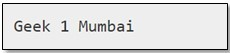# Convert JSON data Into a Custom Python Object

Let us see how to convert JSON data into a custom object in Python. Converting JSON data into a custom python object is also known as decoding or deserializing JSON data. To decode JSON data we can make use of the json.loads(), json.load() method and the object_hook parameter. The object_hook parameter is used so that, when we execute json.loads(), the return value of object_hook will be used instead of the default dict value.We can also implement custom decoders using this.
Example 1 :

## Python3

 `# importing the module` `import` `json` `from` `collections ``import` `namedtuple`   `# creating the data` `data ``=` `'{"name" : "Geek", "id" : 1, "location" : "Mumbai"}'`   `# making the object` `x ``=` `json.loads(data, object_hook ``=` `               ``lambda` `d : namedtuple(``'X'``, d.keys())` `               ``(``*``d.values()))`   `# accessing the JSON data as an object` `print``(x.name, x.``id``, x.location)`

Output :As we can see in the above example, the namedtuple is a class, under the collections module. It contains keys that are mapped to some values. In this case, we can access the elements using keys and indexes. We can also create a custom decoder function, in which we can convert dict into a custom Python type and pass the value to the object_hook parameter which is illustrated in the next example.
Example 2 :

## Python3

 `# importing the module` `import` `json` `from` `collections ``import` `namedtuple`   `# customDecoder function` `def` `customDecoder(geekDict):` `    ``return` `namedtuple(``'X'``, geekDict.keys())(``*``geekDict.values())`   `# creating the data` `geekJsonData ``=` `'{"name" : "GeekCustomDecoder", "id" : 2, "location" : "Pune"}'`   `# creating the object` `x ``=` `json.loads(geekJsonData, object_hook ``=` `customDecoder)`   `# accessing the JSON data as an object` `print``(x.name, x.``id``, x.location)`

Output :We can also use SimpleNamespace class from the types module as the container for JSON objects. Advantages of a SimpleNamespace solution over a namedtuple solution: –

1. It is faster because it does not create a class for each object.
2. It is shorter and simpler.

Example 3 :

## Python3

 `# importing the module` `import` `json` `try``:` `    ``from` `types ``import` `SimpleNamespace as Namespace` `except` `ImportError:` `    ``from` `argparse ``import` `Namespace`   `# creating the data` `data ``=` `'{"name" : "GeekNamespace", "id" : 3, "location" : "Bangalore"}'`   `# creating the object` `x ``=` `json.loads(data, object_hook ``=` `lambda` `d : Namespace(``*``*``d))`   `# accessing the JSON data as an object` `print``(x.name, x.``id``, x.location)`

Output :